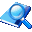﻿ qrjxx - 取软件信息万捷网络验证系统在线帮助文档
Help > WebApi接口说明 >
qrjxx - 取软件信息

### 接口原型：

\$data = qrjxx([软件编号],[信息值]);//返回软件的配置信息，多个值之间用<|>分隔

接口名称  说明
qrjxx 根据软件编号返回软件的配置信息
参数  说明

1 = 最新版本

2  = 公告

3 =  更新地址

4 = 服务器时间戳

5 =  更新信息

6 = 是否强制更新（yes /no）

7 = 自助解绑是否开启（yes/no）

8 =  换机扣除的分钟数

9 = 登录方式(0=禁止，1=不绑定登录，2= 绑定登录)

10 = 循环检测分钟数

11 = 注册方式（0=禁止注册，1=开放注册，2= 带卡注册）

12 = 允许几开

13 = 免费解绑次数

14~17 = 系统保留

18 = 自定义信息

19 = 链接1标题

20 = 链接1网址

21 =  链接2标题

22 = 链接2网址

23 = 链接3标题

24 = 链接3网址

25 = 链接4标题

26 = 链接4网址

（多个信息值用小写逗号隔开，如：1,2,3,4）

### 访问示例：

http://服务器地址/api.php?name=接口名称&c1=软件编号&c2=1,3,5

### 在WebApi接口中的调用方法（将下面的代码复制到后台的WebApi代码框中）：

 function  zdy_ApiName(\$rjbh,\$xxz){//请将 ApiName 改为您自己想要定义的任意名称 \$data=qrjxx(\$rjbh,\$xxz); return \$data;}